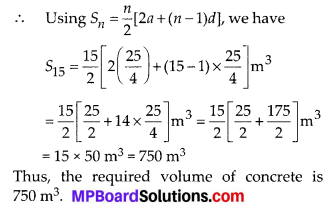# MP Board Class 10th Maths Solutions Chapter 5 Arithmetic Progressions Ex 5.4

In this article, we will share MP Board Class 10th Maths Book Solutions Chapter 5 Arithmetic Progressions Ex 5.4 Pdf, These solutions are solved subject experts from the latest edition books.

## MP Board Class 10th Maths Solutions Chapter 5 Arithmetic Progressions Ex 5.4Question 1.
Which term of the AP : 121,117,113, ……., is its first negative term? [Hint: Find n for an < 0]
Solution:
We have the A.P. having a = 121 and d = 117 – 121 = -4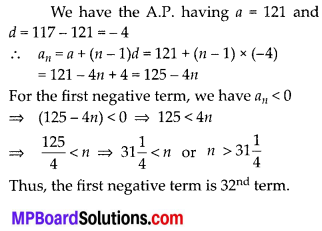Thus, the first negative term is 32nd term.Question 2.
The sum of the third and the seventh terms of an AP is 6 and their product is 8. Find the sum of first sixteen terms of the AP
Solution: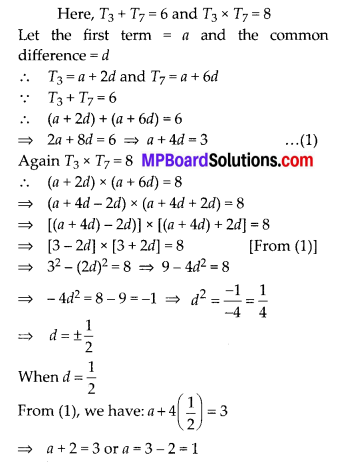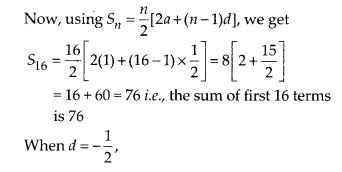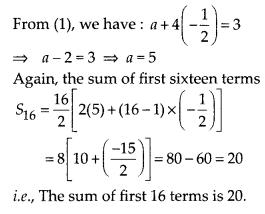Question 3.
A ladder has rungs 25 cm apart (see figure).The rungs decrease uniformly in length from 45 cm at the bottom to 25 cm at the top. If the top and the bottom rungs are $$2 \frac{1}{2}$$ m apart, what is the length of the wood required for the rungs? 250
[Hint: Number of rungs = $$\frac{250}{25}+1$$]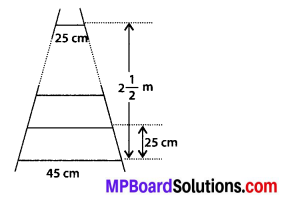Solution: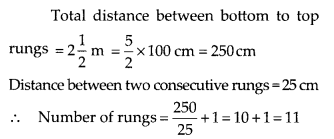Length of the 1st rung (bottom rung) = 45 cm
Length of the 11th rung (top rung) = 25 cm
Let the length of each successive rung decreases by x cm.
∴ Total length of the rungs = 45 cm + (45 – x) cm + (45 – 2x) cm + ….. + 25 cm
Here, the number 45, (45 – x), (45 – 2x), …., 25 are in an AP such that
First term (a) = 45
and last term (l) = 25
Number of terms, n = 11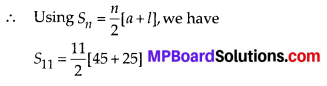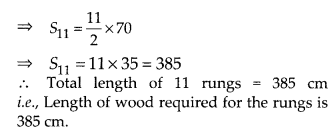Question 4.
The houses of a row are numbered consecutively from 1 to 49. Show that there is a value of x such that the sum of the number of the houses preceding the house numbered x is equal to the sum of the number of the houses following it. Find this value of x. [Hint: Sx-1 = S49 – Sx]
Solution:
We have the following consecutive numbers on the houses of a row; 1, 2, 3, 4, 5, ….. , 49.
These numbers are in AP, such that a = 1, d = 2 – 1 = 1, n = 49
Let one of the houses be numbered as x.
∴ Number of houses preceding it = x – 1
Number of houses following it = 49 – x
Now, the sum of the house-numbers preceding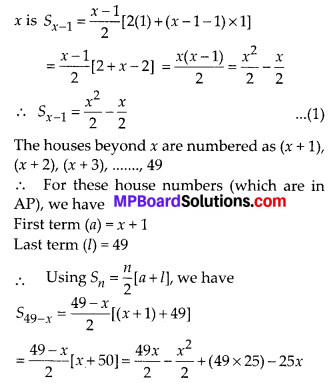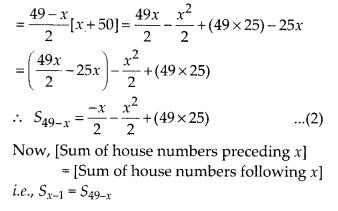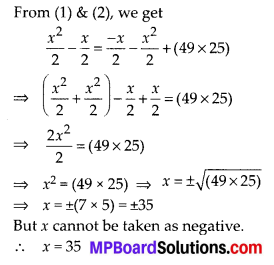Question 5.
A small terrace at a football ground comprises of 15 steps each of which is 50 m long and built of solid concrete. Each step has a rise of $$\frac{1}{4}$$m and a tread of $$\frac{1}{2}$$m (see fig.) Calculate the total volume of concrete required to build the terrace.
[Hint: Volume of concrete required to build the 1st step $$\frac{1}{4} \times \frac{1}{2} \times 50 m^{3}$$]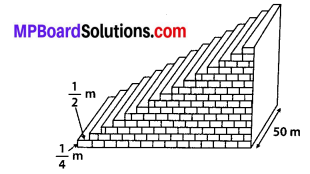Solution: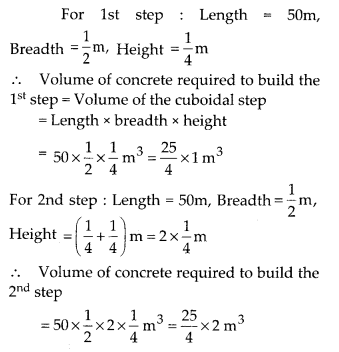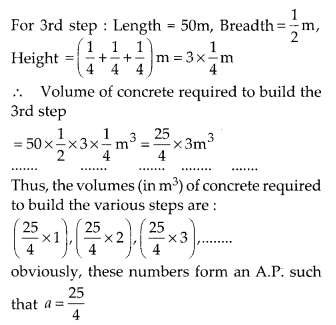$$d=\frac{25}{2}-\frac{25}{4}=\frac{25}{4}$$
Here, total number of steps n = 15
Total volume of concrete required to build 15 steps is given by the sum of their individual volumes.